• 这篇文章主要介绍了opencv python如何实现图像二值化,文中通过示例代码介绍的非常详细，对大家的学习或者工作具有一定的参考学习价值,需要的朋友可以参考下 代码如下 import cv2 as cv import numpy as np import ...
• 图像二值化处理时图像处理过程中非常常见的一种操作，在python中比较常用的主要是opencv,pil两个第三库，对比来看的话，opencv使用要更加的方便灵活， 文本主要介绍以下基于opencv的图像二值化处理方法。 首相我们...
      图像二值化处理时图像处理过程中非常常见的一种操作，在python中比较常用的主要是opencv,pil两个第三库，对比来看的话，opencv使用要更加的方便灵活，
文本主要介绍以下基于opencv的图像二值化处理方法。
首先我们来看一种比较简单的图像二值化处理方法。

全局阈值二值化

主要思路就是设置一个阈值，低于该阈值的像素置为0(黑色)，高于该阈值的像素置为255(白色)，下面来看代码

def image_binarization(img):
# 将图片转为灰度图
gray = cv2.cvtColor(img, cv2.COLOR_BGR2GRAY)

# retval, dst = cv2.threshold(gray, 110, 255, cv2.THRESH_BINARY)
# 最大类间方差法(大津算法)，thresh会被忽略，自动计算一个阈值
retval, dst = cv2.threshold(gray, 127, 255, cv2.THRESH_BINARY)
cv2.imwrite('binary.jpg', dst)

cv2.threshold(src, thresh, maxval, type)

src：表示的是图片源
thresh：表示的是阈值（分割值）
maxval：表示的是最大值
type：表示的是这里划分的时候使用的是什么类型的算法，包含以下几种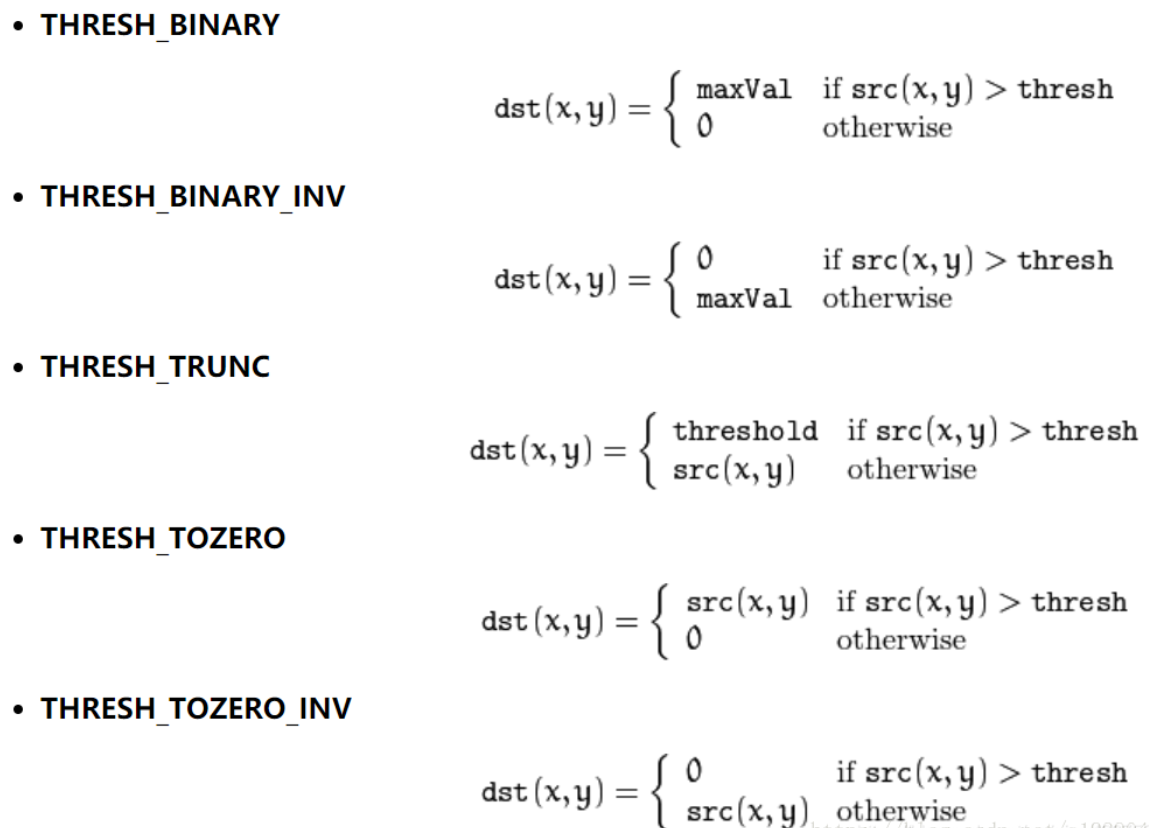该函数有两个返回值，第一个retVal（得到的阈值），第二个就是阈值化后的图像。

上述方法较为简单，且运算效率较高，但是存在一个问题，如果你想批量处理一批文本图片，有的图片文本较深，有的图片文本较浅，那么你设置一个单一的阈值就会存在问题，如果阈值较小，那么颜色较浅的文本图片二值化过后内容会丢失，如果阈值较大，图片二值化处理后，文本内容很可能和背景融为一体较难区分。

阈值设置为70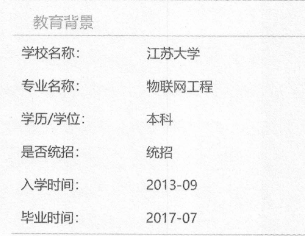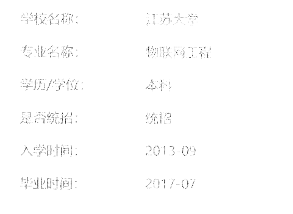处理前                                                                   处理后

阈值设置为110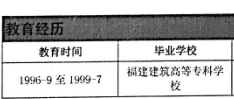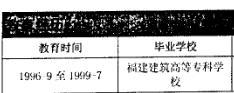处理前                                                                   处理后

大津算法cv2.threshold处理上述5种算子之外，还提供了一种可以根据图片像素自动计算阈值的一种方法，cv2.THRESH_OTSU(最大类间方差法，也叫大津算法），thresh会被忽略，自动计算一个阈值。

 retval, dst = cv2.threshold(gray, 0, 255,  cv2.THRESH_BINARY | cv2.THRESH_OTSU)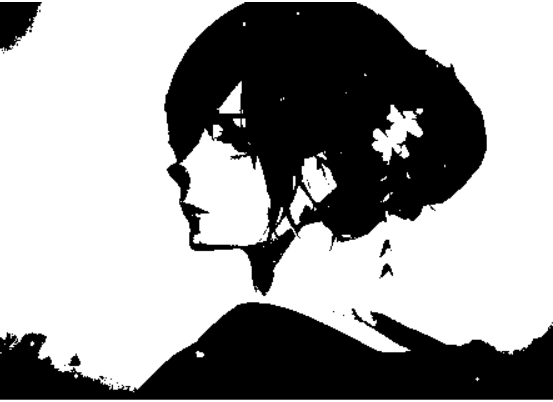处理前                                                                  处理后

虽然该算子可以在一定程度上可以解决上述问题，但是该算子对于比较复杂的图片处理效果还是很不理想，尤其是一些明暗差异较大的图片，下面记录一种可以局部自适应的二值化的方法

局部阈值二值化

为了解决我们上述所说的问题，我们可以采用cv2.adaptiveThreshold方法进行二值化处理，函数的大致意思是以图片中的每个像素点为中心取他周围n*n的范围内的像素，然后根据这个区域内的像素值来计算出来一个阈值，决定当前像素点是处理成0还是255。

主要包含以下几个步骤

1. 加载图像
2. 转灰度图
3. 图像预处理（根据个人需求进行处理）
4. 二值化处理
5. 降噪(根据个人需求进行处理)

def image_binarization_part_situation(img):
'''
局部二值化
:return:
'''
# 转灰度图
gray = cv2.cvtColor(img, cv2.COLOR_BGR2GRAY)
# 图像压缩(非必要步骤)
new_gray = np.uint8((255 * (gray/255.0)**1.4))
# 二值化
dst = cv2.adaptiveThreshold(new_gray, 255, cv2.ADAPTIVE_THRESH_GAUSSIAN_C, cv2.THRESH_BINARY, 15, 1)
# 中值滤波
img_median = cv2.medianBlur(dst, 5)


核心函数

cv2.adaptiveThreshold(src, maxValue, adaptiveMethod, thresholdType, blockSize, C, dst=None)

src：需要进行二值化的一张灰度图像

maxValue：满足条件的像素点需要设置的灰度值。（将要设置的灰度值）

adaptiveMethod：自适应阈值算法。可选ADAPTIVE_THRESH_MEAN_C 或 ADAPTIVE_THRESH_GAUSSIAN_C

thresholdType：opencv提供的二值化方法，只能THRESH_BINARY或者THRESH_BINARY_INV

blockSize：要分成的区域大小，上面的N值，取奇数

C：常数，每个区域计算出的阈值的基础上在减去这个常数作为这个区域的最终阈值，可以为负数

dst：输出图像，可以忽略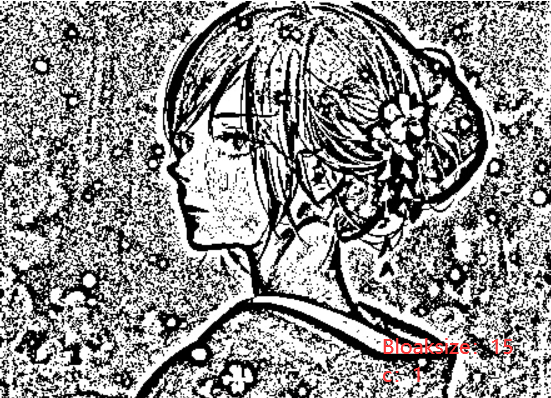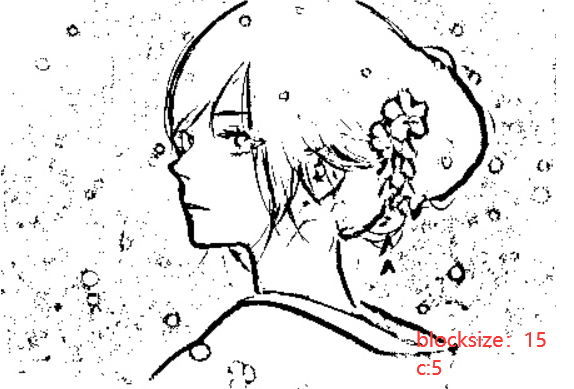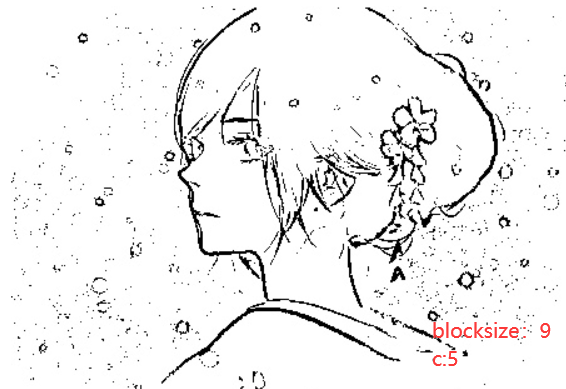展开全文opencv 计算机视觉
• 在本文中，我们学习如何使用 NumPy 对图像进行二值化，当然，我们使用 OpenCV 来读取灰度和 RGB 格式的图像。 要理解二进制是什么ーー二进制是由两种东西组成的东西。在计算机术语中，二进制只是0和1。如果我们...
在本文中，我们将学习如何使用 NumPy 对图像进行二值化，当然，我们将使用 OpenCV 来读取灰度和 RGB 格式的图像。

要理解二进制是什么ーー二进制是由两种东西组成的东西。在计算机术语中，二进制只是0和1。如果我们要把同样的事情在图像中联系起来，那么就是说黑白图像中：

0 表示黑色

1 表示白色

在学习图像处理的初始阶段，我们通常认为灰度图像是一个二值图像。虽然不是。但是慢慢地，当我们开始谈论这个话题时，我们意识到我们错得有多离谱。因此，接下来，我们将学习如何使用库和不使用库(NumPy 用于矩阵操作，只是为了避免使用规则 for 循环时程序速度缓慢)将图像进行二进制化。除此之外，我们还将利用 Matplotlib 来绘制结果。

RGB 和灰度概述

对于灰度图像来说，二值化运算的效果非常好。彩色(RGB)图像的问题在于，每个像素都是一个矢量，代表3个唯一的值，一个是红色，一个是绿色，一个是蓝色。

一个典型的灰度图像的矩阵看起来像：

array([[162, 162, 162, ..., 170, 155, 128],       [162, 162, 162, ..., 170, 155, 128],       [162, 162, 162, ..., 170, 155, 128],       ...,       [ 43,  43,  50, ..., 104, 100,  98],       [ 44,  44,  55, ..., 104, 105, 108],       [ 44,  44,  55, ..., 104, 105, 108]], dtype=uint8)

一个典型的 RGB 图像的矩阵看起来像：

array([[[226, 137, 125], ..., [200,  99,  90]],       [[226, 137, 125], ..., [200,  99,  90]],       [[226, 137, 125], ..., [200,  99,  90]],       ...,       [[ 84,  18,  60], ..., [177,  62,  79]],       [[ 82,  22,  57], ..., [185,  74,  81]],       [[ 82,  22,  57], ..., [185,  74,  81]]], dtype=uint8)

如果我们将 R，G 和 B 三个通道的像素从上面的矩阵中分离出来，我们得到。

R 矩阵

array([[226, 226, 223, ..., 230, 221, 200],       [226, 226, 223, ..., 230, 221, 200],       [226, 226, 223, ..., 230, 221, 200],       ...,       [ 84,  84,  92, ..., 173, 172, 177],       [ 82,  82,  96, ..., 179, 181, 185],       [ 82,  82,  96, ..., 179, 181, 185]], dtype=uint8)

G 矩阵​​​​​​​

array([[137, 137, 137, ..., 148, 130,  99],       [137, 137, 137, ..., 148, 130,  99],       [137, 137, 137, ..., 148, 130,  99],       ...,       [ 18,  18,  27, ...,  73,  68,  62],       [ 22,  22,  32, ...,  70,  71,  74],       [ 22,  22,  32, ...,  70,  71,  74]], dtype=uint8)

B 矩阵​​​​​​​

array([[125, 125, 133, ..., 122, 110,  90],       [125, 125, 133, ..., 122, 110,  90],       [125, 125, 133, ..., 122, 110,  90],       ...,       [ 60,  60,  58, ...,  84,  76,  79],       [ 57,  57,  62, ...,  79,  81,  81],       [ 57,  57,  62, ...,  79,  81,  81]], dtype=uint8)

无论我们对灰度图像进行什么操作，我们都需要对 RGB 图像进行相同的计算，但需要将 R，G 和 B 通道分离3次，最后将它们合并为一个正确的 RGB 图像。

编程时间

我们主要使用的软件库是：

NumPy

Matplotlib

OpenCV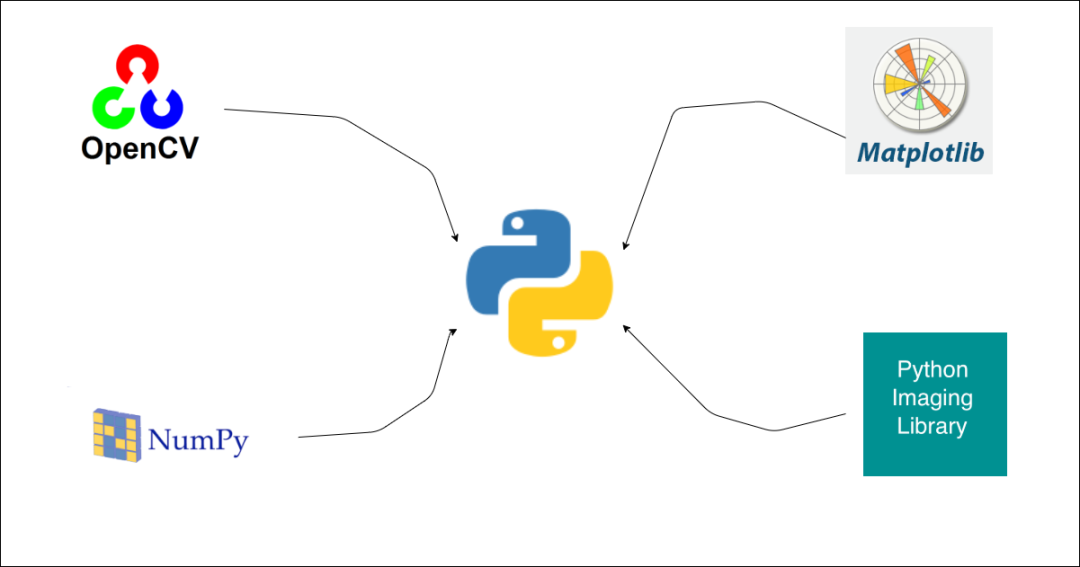导入软件库​​​​​​​

import numpy as npimport cv2import jsonfrom matplotlib import pyplot as plt

读取图片​​​​​​​

def read_this(image_file, gray_scale=False):    image_src = cv2.imread(image_file)    if gray_scale:        image_src = cv2.cvtColor(image_src, cv2.COLOR_BGR2GRAY)    else:        image_src = cv2.cvtColor(image_src, cv2.COLOR_BGR2RGB)    return image_src

上面的函数以灰度或者 RGB 的形式读取图像并返回图像矩阵。

实现代码

为了将图像转换为二值图像，我们可以简单地利用 cv2库中提供的threshold()方法。这种方法，不管图像是什么(灰度或 RGB)转换成二进制。使用时需要4个参数。

src：基本上就是图像矩阵

thresh：阈值，基于这个阈值像素被赋予一个新的值。如果像素小于这个值，我们将把这些像素重新赋值为255；否则，像素将重新定值为0

maxval：图像可以包含的最大像素值(255)

type：一种给定的阈值类型，并基于该类型计算操作。

在此之后，我们将在下面的函数绘制结果以查看变化。​​​​​​​

def binarize_lib(image_file, thresh_val=127, with_plot=False, gray_scale=False):    image_src = read_this(image_file=image_file, gray_scale=gray_scale)    th, image_b = cv2.threshold(src=image_src, thresh=thresh_val, maxval=255, type=cv2.THRESH_BINARY)    if with_plot:        cmap_val = None if not gray_scale else 'gray'        fig, (ax1, ax2) = plt.subplots(nrows=1, ncols=2, figsize=(10, 20))                ax1.axis("off")        ax1.title.set_text('Original')                ax2.axis("off")        ax2.title.set_text("Binarized")                ax1.imshow(image_src, cmap=cmap_val)        ax2.imshow(image_b, cmap=cmap_val)        return True    return image_b

让我们测试一下上面的函数：

binarize_lib(image_file='lena_original.png', with_plot=True)binarize_lib(image_file='lena_original.png', with_plot=True, gray_scale=True)现在我们已经看到了原始图像和二进制图像的结果，很明显，使用库中提供的函数编写的代码对这两者都适用。是时候让我们从头开始编写如何使用 NumPy 对图像进行二值化。

从零开始的代码实现

首先，我们将编写一个函数，将小于指定阈值的像素值重新赋值为255。

通过这样做，我们可以看到下面这样的东西：​​​​​​​

def convert_binary(image_matrix, thresh_val):    white = 255    black = 0        initial_conv = np.where((image_matrix <= thresh_val), image_matrix, white)    final_conv = np.where((initial_conv > thresh_val), initial_conv, black)        return final_conv

我们将上面的函数通过分离 r、 g 和 b 值进行三次调用，最后将它们合并得到二值化图像。一旦这样做，我们就可以像以前那样绘制结果。​​​​​​​

def binarize_this(image_file, thresh_val=127, with_plot=False, gray_scale=False):    image_src = read_this(image_file=image_file, gray_scale=gray_scale)        if not gray_scale:        cmap_val = None        r_img, g_img, b_img = image_src[:, :, 0], image_src[:, :, 1], image_src[:, :, 2]                r_b = convert_binary(image_matrix=r_img, thresh_val=thresh_val)        g_b = convert_binary(image_matrix=g_img, thresh_val=thresh_val)        b_b = convert_binary(image_matrix=b_img, thresh_val=thresh_val)                image_b = np.dstack(tup=(r_b, g_b, b_b))    else:        cmap_val = 'gray'        image_b = convert_binary(image_matrix=image_src, thresh_val=thresh_val)        if with_plot:        fig, (ax1, ax2) = plt.subplots(nrows=1, ncols=2, figsize=(10, 20))                ax1.axis("off")        ax1.title.set_text('Original')                ax2.axis("off")        ax2.title.set_text("Binarized")                ax1.imshow(image_src, cmap=cmap_val)        ax2.imshow(image_b, cmap=cmap_val)        return True    return image_b

我们只是使用 NumPy 创建了我们的二进制化代码：

binarize_this(image_file='lena_original.png', with_plot=True)binarize_this(image_file='lena_original.png', with_plot=True, gray_scale=True)至此，我们发现使用 NumPy 从零编写的结果与我们调用库函数编写的代码得到的结果非常相似。

展开全文opencv java numpy 计算机视觉
• 二值化2.1.取中间阀值1272.2.取所有像素点灰度的平均值3.灰度变换3.1.反相3.2.像素值变换到100~200之间3.3.像素值求平方，使较暗的像素值变得更小3.4.灰度变换函数对比 原图如下 1.灰度化 参考《Python...
Python图像处理（一）【灰度化、二值化、灰度变换】1.灰度化1.1浮点算法1.2.整数算法1.3.平均值法1.4.移位法1.5.单通道法（只取绿色通道）2.二值化2.1.取中间阀值1272.2.取所有像素点灰度的平均值3.灰度变换3.1.反相3.2.将像素值变换到100~200之间3.3.将像素值求平方，使较暗的像素值变得更小3.4.灰度变换函数对比
原图如下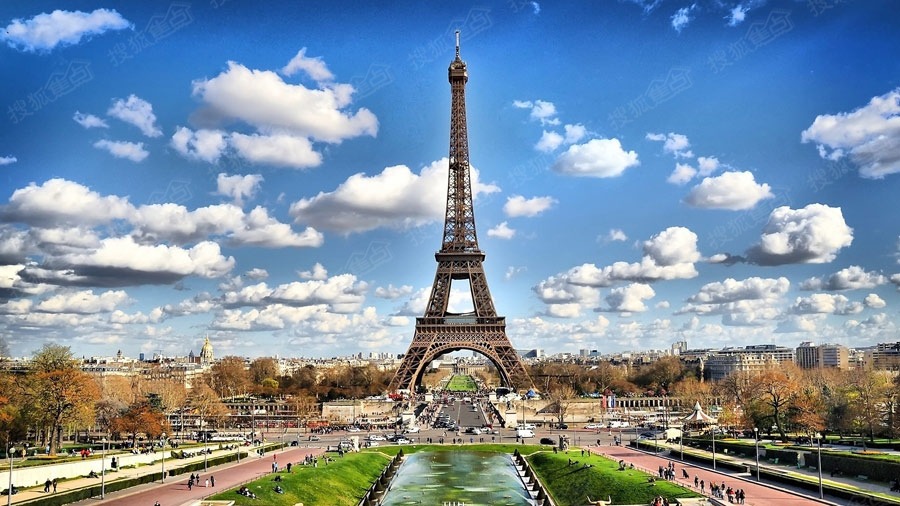1.灰度化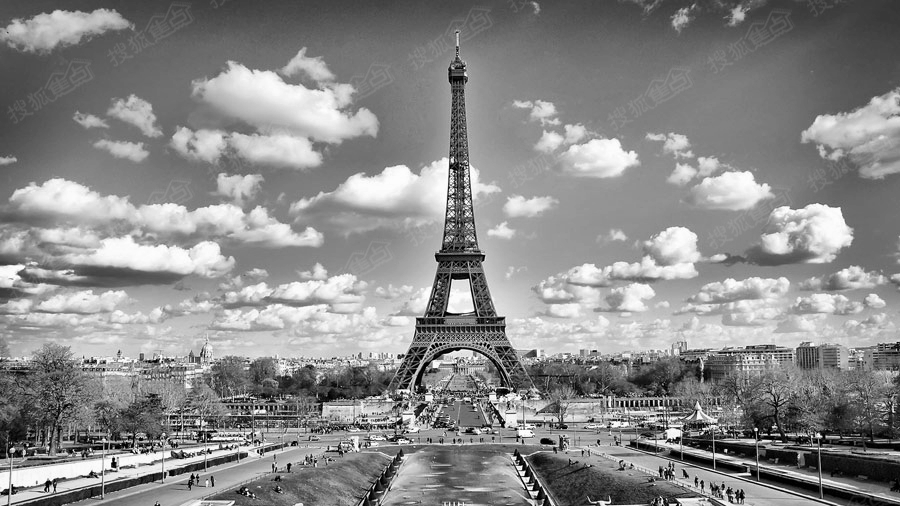参考《Python图像灰度变换及图像数组操作》实现以下几种灰度化

获取图片及显示图片的通用方法如下：
from PIL import Image
import matplotlib.pyplot as plt
import numpy as np

#获取图片
def getimg():
return Image.open("f:/pic/timg.jpg")

#显示图片
def showimg(img, isgray=False):
plt.axis("off")
if isgray == True:
plt.imshow(img, cmap='gray')
else:
plt.imshow(img)
plt.show()

PIL库自带方法实现灰度化
im = getimg()
im_gray = im.convert('L')
showimg(im_gray, True)

1.1浮点算法
im = getimg()
im = np.array(im)
im[:,:,0] = im[:,:,0]*0.3
im[:,:,1] = im[:,:,1]*0.59
im[:,:,2] = im[:,:,2]*0.11
im = np.sum(im, axis=2)
showimg(Image.fromarray(im), True)

1.2.整数算法
im1 = getimg()
#创建数组时指定数据类型，否则默认uint8乘法运算会溢出
im1 = np.array(im1, dtype=np.float32)
im1[...,0] = im1[...,0]*30.0
im1[...,1] = im1[...,1]*59.0
im1[...,2] = im1[...,2]*11.0
im1 = np.sum(im1, axis=2)
im1[...,:] = im1[...,:]/100.0
showimg(Image.fromarray(im1), True)

1.3.平均值法
im2 =  getimg()
im2 = np.array(im2, dtype=np.float32)
im2 = np.sum(im2, axis=2)
im2[...,:] = im2[...,:]/3.0
showimg(Image.fromarray(im2), True)

1.4.移位法
im3 = getimg()
im3 = np.array(im3, dtype=np.int32)
im3[...,0] = im3[...,0]*28.0
im3[...,1] = im3[...,1]*151.0
im3[...,2] = im3[...,2]*77.0
im3 = np.sum(im3, axis=2)

arr = [np.right_shift(y.item(), 8) for x in im3 for y in x]
arr = np.array(arr)
arr.resize(im3.shape)
showimg(Image.fromarray(arr), True)

1.5.单通道法（只取绿色通道）
im4 = getimg()
im4 = np.array(im4, dtype=np.int32)
im4[...,0] = 0
im4[...,2] = 0
im4 = np.sum(im4, axis=2)
showimg(Image.fromarray(im4), True)

2.二值化
2.1.取中间阀值127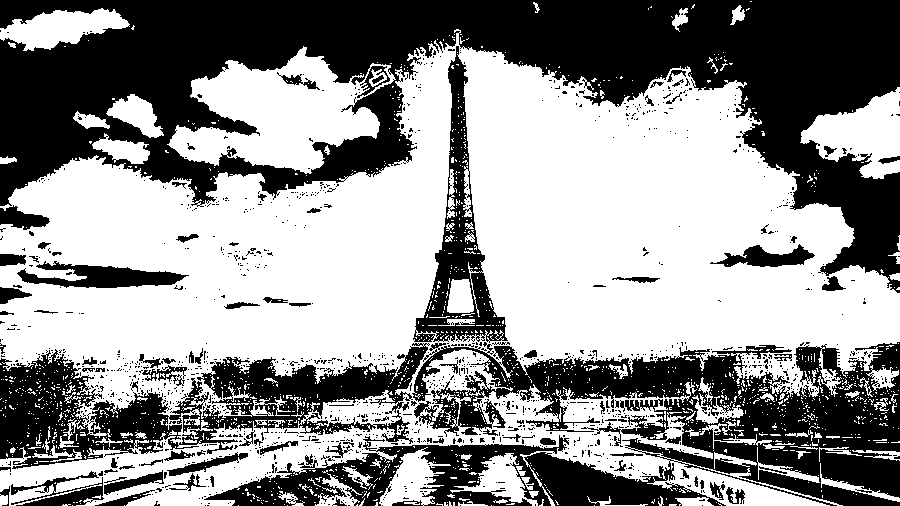im5 = getimg()
im5 = np.array(im5.convert('L'))
im5 = np.where(im5[...,:] < 127, 0, 255)
showimg(Image.fromarray(im5), True)

2.2.取所有像素点灰度的平均值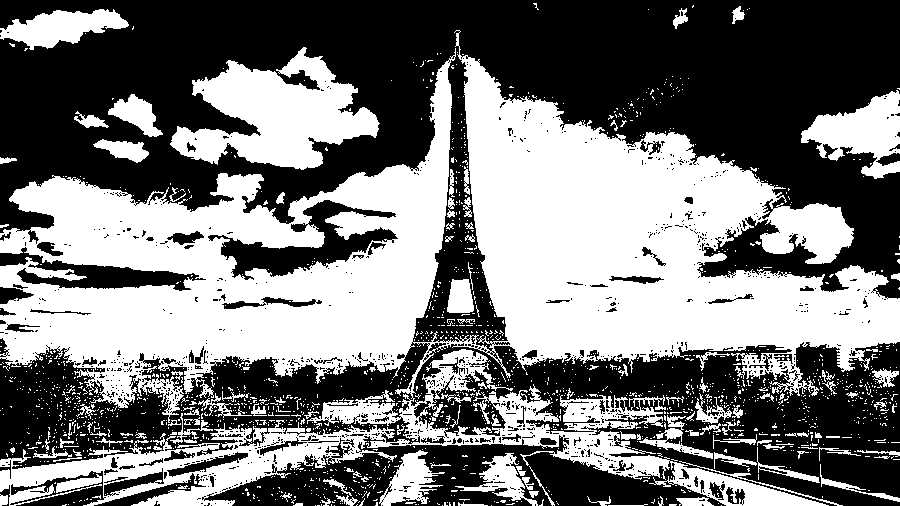im = getimg()
im_gray1 = im.convert('L')
im_gray1 = np.array(im_gray1)
avg_gray = np.average(im_gray1)
im_gray1 = np.where(im_gray1[...,:] < avg_gray, 0, 255)
showimg(Image.fromarray(im_gray1), True)

3.灰度变换
3.1.反相im = getimg()
im_gray = im.convert('L')
im_arr = np.array(im_gray)
im1 = 255 - im_arr
showimg(Image.fromarray(im1))

3.2.将像素值变换到100~200之间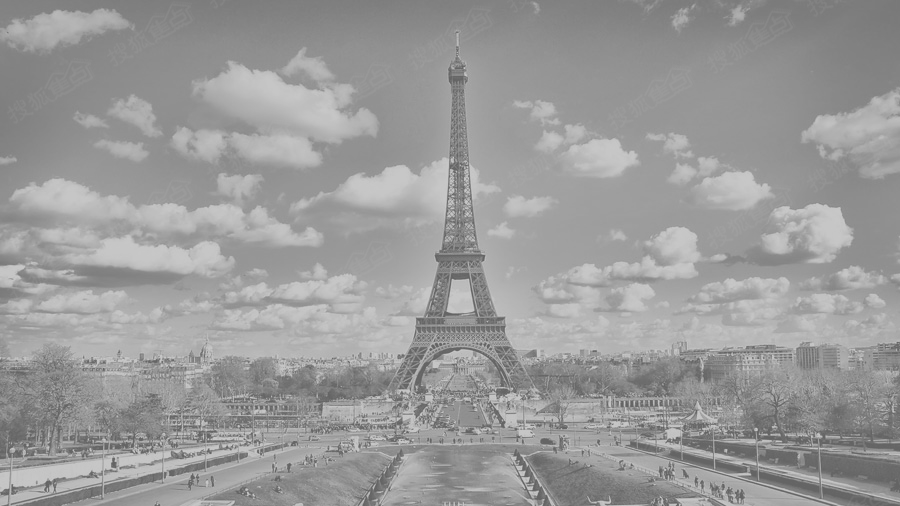im = getimg()
im_gray = im.convert('L')
im2 = (100.0/255)*im_gray +100
showimg(Image.fromarray(im2))

3.3.将像素值求平方，使较暗的像素值变得更小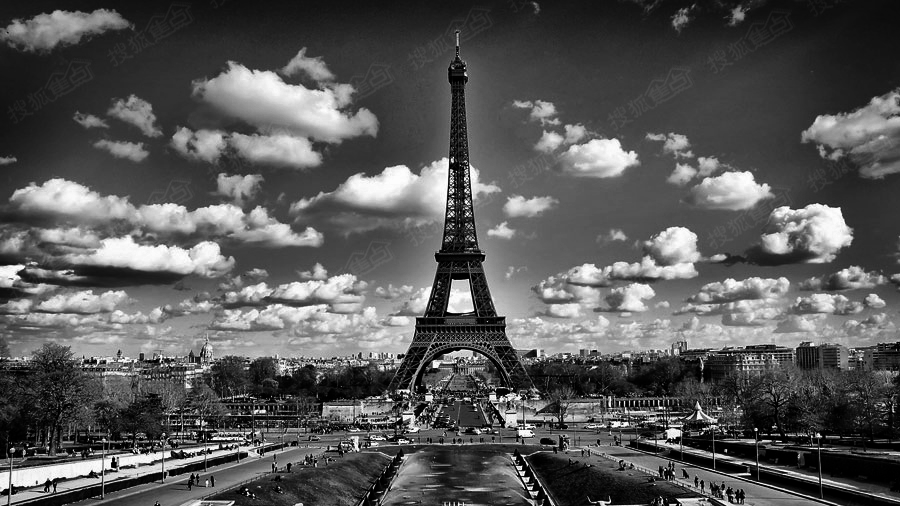im = getimg()
im_gray = im.convert('L')
im3 = 255.0*(im_gray /255.0)**2
showimg(Image.fromarray(im3))

3.4.灰度变换函数对比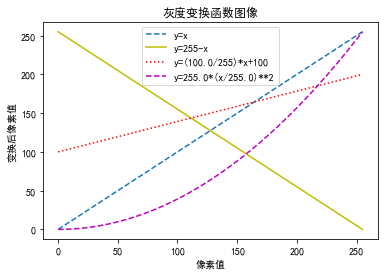plt.rcParams['font.sans-serif'] = ['SimHei']
plt.title('灰度变换函数图像')
plt.xlabel('像素值')
plt.ylabel('变换后像素值')

x1 = np.arange(0, 256)
y1 = np.arange(0, 256)

f1, = plt.plot(x1, y1, '--')

y2 = 255 - x1
f2, = plt.plot(x1, y2, 'y')

y3 = (100.0/255)*x1 + 100
f3, = plt.plot(x1, y3, 'r:')

y4 = 255.0*(x1/255.0)**2
f4, = plt.plot(x1, y4, 'm--')
plt.legend((f1, f2, f3, f4), ('y=x','y=255-x','y=(100.0/255)*x+100','y=255.0*(x/255.0)**2'),loc='upper center')
plt.show()



展开全文• 本篇介绍彩色图像灰度化和二值化的原理，并使用Python来实现对应算法内容。


本篇将介绍彩色图像灰度化和二值化的原理，并使用Python来实现对应算法内容。

[彩色图像灰度化]

1.定义

在上一节中，我们介绍了8位单色灰度图，它使用0-255来表示一个像素，但在实际使用中，我们最常用的还是彩色图像灰度化。对于32位bgra彩色图像，或者24位rgb/bgr彩色图像，一个像素由红绿蓝三原色混合而成，这也就是绘画中的调色过程，如何调制灰色？其实很简单，只要红绿蓝以相同程度进行混合，那么结果就呈现出灰色。基于这个原理，我们可以给出彩色图像灰度化的本质：R=G=B，即红绿蓝三通道的像素值相等，此时，彩色图就表现为灰度图，而这个过程，就叫做彩色图像的灰度化。

如图Fig.1所示，左侧位32bgra彩色图，右侧为对应的灰度图，该灰度图算法来自Photoshop“去色”命令。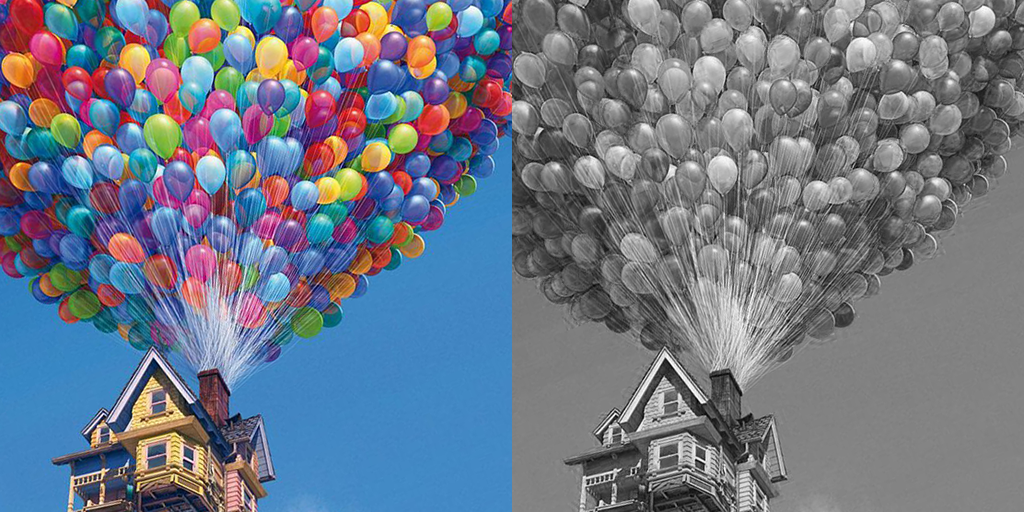(a)32位彩色图                                  (b)32位灰度图

Fig.1 彩色图像灰度化示例

2.算法

彩色图像灰度化的算法公式一般常用的有三种：明度公式，视觉公式和Photoshop去色公式。

①明度公式

明度公式，实际上就是取一个像素的红绿蓝三通道均值，将均值作为该像素的灰度值，以此实现灰度化效果。我们用Python语言实现，代码如下：

///

import numpy as np
import  matplotlib.pyplot as plt
import matplotlib.image as img

#明度法图像灰度化
def imgGray_Lum(im):
"""
image gray
im: source image
Return gray image.
"""
#imgarray = np.array(im)
imgarray = np.array(im, dtype=np.float32)#以浮点型读取图像数据
rows = im.shape
cols = im.shape
for i in range(rows):
for j in range(cols):
imgarray[i, j, :] = np.clip((imgarray[i, j, 0] + imgarray[i, j, 1] + imgarray[i, j, 2]) * 0.3333,0.0,255.0)
return imgarray.astype(np.uint8)#保存为uint8类型图像

im = img.imread("test.jpg")#图像读取
im = imgGray_Lum(im)
plt.imshow(im)#图像显示
img.imsave('save.jpg',im)

我们给出明度法彩色图像灰度化的效果测试，如图Fig.2所示。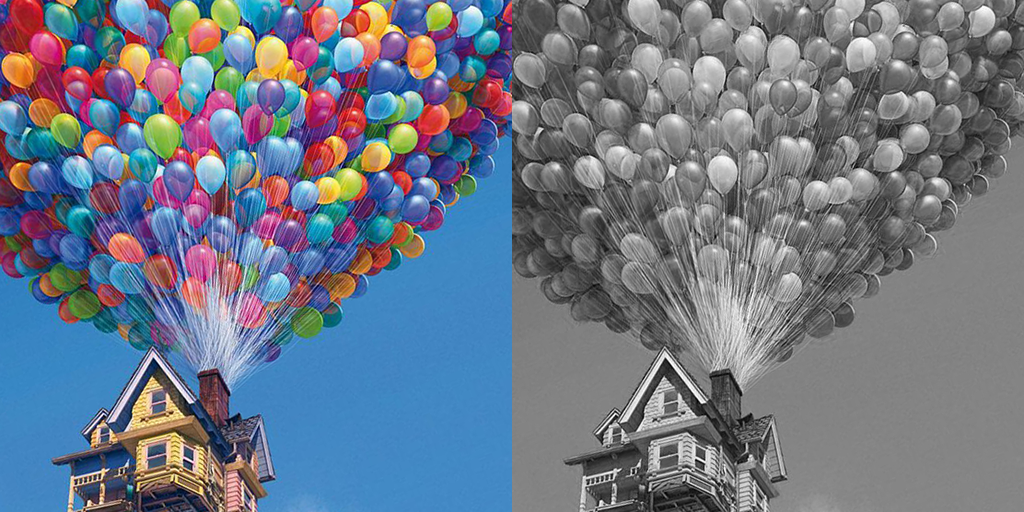Fig.2彩色图像灰度化(明度公式)

②视觉公式

由于人眼对于颜色的感应是不同的，人眼对绿色的敏感最高，对蓝色敏感最低，因此，上述公式是通过对像素RGB三分量进行加权平均，得到一种较合理的灰度图像，该公式也是最经典的灰度化公式。

我们用Python语言实现，代码如下：

/

import numpy as np
import  matplotlib.pyplot as plt
import matplotlib.image as img

#视觉法图像灰度化
def imgGray_Version(im):
"""
image gray
im: source image
Return gray image.
"""
imgarray = np.array(im, dtype = np.float32)
rows = im.shape
cols = im.shape
for i in range(rows):
for j in range(cols):
imgarray[i, j, :] = (imgarray[i, j, 0] * 0.299 + imgarray[i, j, 1] * 0.587 + imgarray[i, j, 2] * 0.114)
return imgarray.astype(np.uint8)

im = img.imread("test.jpg")#图像读取
im = imgGray_Version(im)
plt.imshow(im)#图像显示
img.imsave('save.jpg',im)

我们给出视觉颜色法彩色图像灰度化的效果测试，如图Fig.3所示。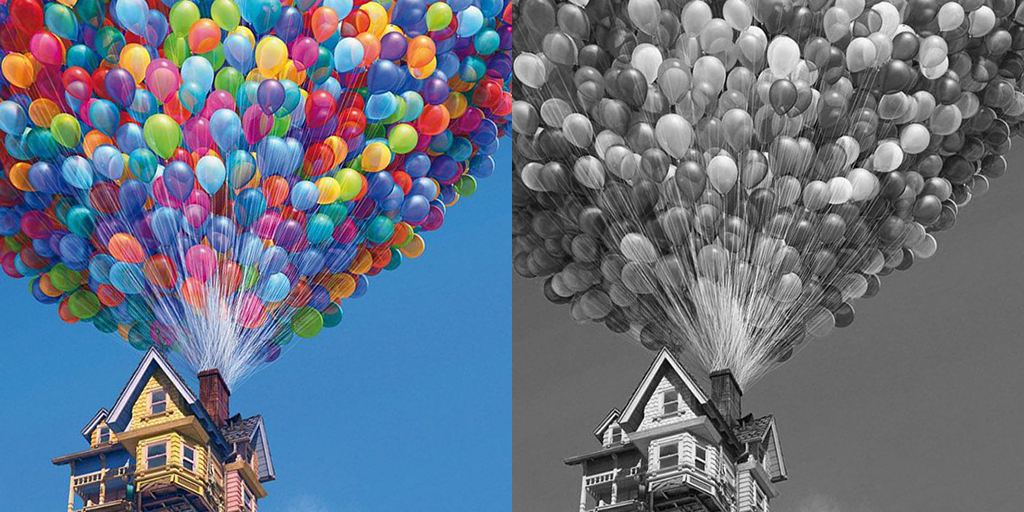Fig.3 彩色图像灰度化(视觉颜色公式)

③Photoshop去色公式

Photoshop中的去色公式，是一种考虑了图像对比度信息的灰度化公式，可以更好的突出颜色反差，在一些颜色较为接近的图像中表现会比较明显。它的算法比较简单，求取每个像素RGB三通道值的最大值和最小值，然后计算两者的均值，将均值作为灰度化结果即可。我们用Python语言来实现，代码如下：

///

import numpy as np
import  matplotlib.pyplot as plt
import matplotlib.image as img

#Photoshop法图像灰度化
def imgGray_PS(im):
"""
image gray
im: source image
Return gray image.
"""
imgarray = np.array(im, dtype = np.float32)
rows = im.shape
cols = im.shape
for i in range(rows):
for j in range(cols):
maxValue = np.maximum(imgarray[i, j, 0], np.maximum(imgarray[i, j, 1],imgarray[i, j, 2]))
minValue = np.minimum(imgarray[i, j, 0], np.minimum(imgarray[i, j, 1],imgarray[i, j, 2]))
imgarray[i, j, :] = (maxValue+ minValue) * 0.5
return imgarray.astype(np.uint8)

im = img.imread("test.jpg")#图像读取
im = imgGray_PS(im)
plt.imshow(im)#图像显示
img.imsave('save.jpg',im)

我们给出Photoshop去色法彩色图像灰度化的效果测试，如图Fig.4所示。Fig.4 彩色图像灰度化(Photoshop去色法)

上述三种是常用的彩色图像灰度化算法，作为初学者，我们要透过现象看本质，抓住灰度化的本质，那就是：RGB三通道颜色值相同。

灰度化算法并非唯一，大家可以根据自己的需求设计自己的灰度化算法，如此才能举一反三，学以致用。

[彩色图像阈二值化]

作为本小节的第二个内容，彩色图像二值化实际上就是将一副彩色图，转换为只有两种颜色的图像，我们通常说的黑白阈值化只是其中的一个特例，或者说，黑白二值化是狭义的二值化理解，任意两种颜色二值化则是更为宽泛的理解。如图Fig.5所示，这些都叫作二值化。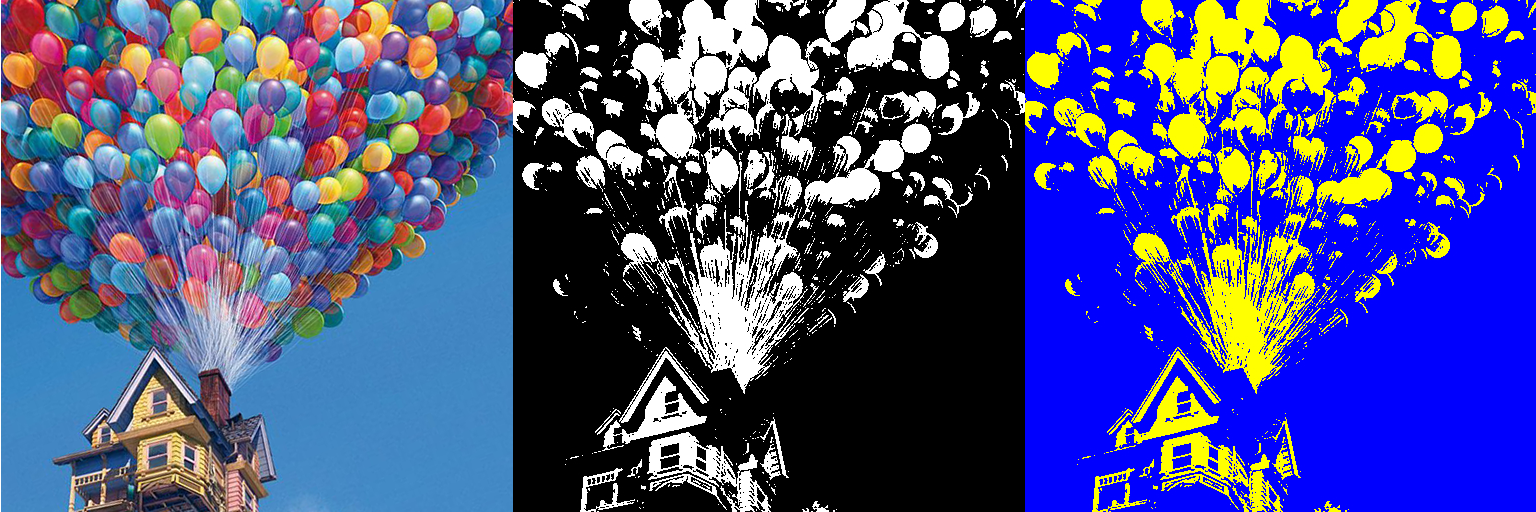Fig.5 彩色图像二值化示例

我们以灰度图像二值化为例，算法公式如下：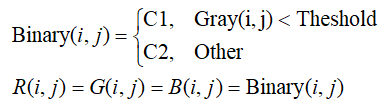其中，C1和C2分别表示两种颜色值，如果为黑白二值化，则C1和C2表示黑白两种颜色，Threshold表示阈值，范围[0,255]，根据阈值将像素划分为C1和C2两类。

我们用Python语言来实现灰度图像二值化算法，代码如下：

import numpy as np
import  matplotlib.pyplot as plt
import matplotlib.image as img

#image binary threshold process
def imgBinaryThreshold(img, threshold=128):
"""
image binary threshold
im: source image
threshold:Threshold from 0 to 255
Return gray image.
"""
imgarray = np.array(im, dtype = np.uint8)
rows = im.shape
cols = im.shape
for i in range(rows):
for j in range(cols):
gray = (imgarray[i, j, 0] * 0.299 + imgarray[i, j, 1] * 0.587 + imgarray[i, j, 2] * 0.114)
if(gray < threshold):
imgarray[i, j, :] = 0
else:
imgarray[i, j, :] = 255
return imgarray.astype(np.uint8)

im = img.imread("test.jpg")#图像读取
im = imgBinaryThreshold(im)
plt.imshow(im)#图像显示
img.imsave('save.jpg',im)

我们给出彩色图像二值化的效果测试，如图Fig.6所示。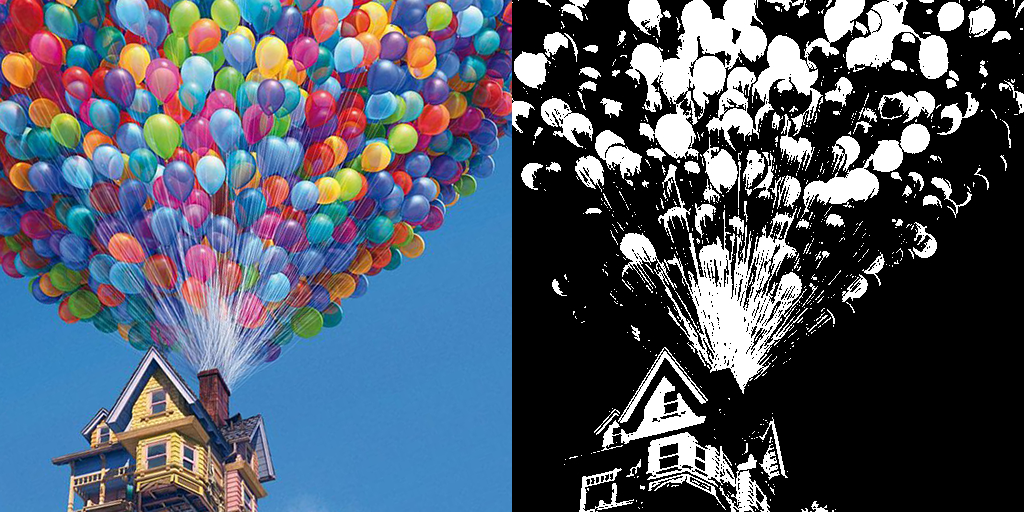Fig.6 彩色图像二值化(黑白二值，阈值Threshold=128)

上述内容就是彩色图像二值化算法，实际上，无论灰度化还是彩色化都并非这么简单，但是作为初学者或者入门者，我们只有掌握了这些最基础的内容，才能去钻研更高深的理论，不积跬步无以至千里就是这个道理。

[知识扩展]

灰度化和二值化都是数字图像处理中很重要的组成部分，灰度化中比较热门的是对比度保留的彩色图像去色算法，研究在特殊图像颜色下的灰度化，如图Fig.7所示，一般的灰度化算法处理之后，图像原有的信息已经无法辨识，而对比度保留的灰度化算法处理结果依然可以清晰保留这些信息。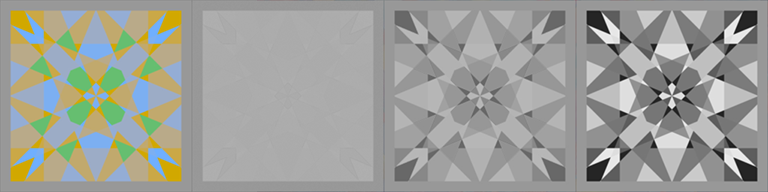(a)原图                              (b)Photoshop灰度化                      (c)Photoshop去色                      (d)对比度保留法

Fig.7 对比度保留法灰度化示例

Fig.5(d)所示方法的相关论文如下：

Color2Gray: Salience-Preserving Color Removal

Contrast Preserving Decolorization

Real-time Contrast Preserving Decolorization

二值化在图像分割中非常常见，二值化的研究难点是如何自适应设置阈值Threshold来将图像二值化处理，常简的一些自动二值化算法有：Ostu阈值化，P分位阈值化，统计阈值化，最大类间方差二值化等等。


展开全文• Python+Opencv 彩色图像二值化 本文转载自：https://blog.csdn.net/what_lei/article/details/49159655 ，侵权删 定义：图像的二值化，就是图像上的像素点的灰度值设置为0或255，也就是整个图像呈现...
• 图像二值化 在图像处理中经常用到二值化，图像的二值化就是图像上的像素点的灰度值设置为0 或255。 主要来讲一下图像二值化的函数 cv2.threshold()函数的作用是一幅灰度图二值化，基本用法如下： cv2....opencv
• 定义：图像二值化，就是将图像上的像素点的灰度值设置为0或255，也就是整个图像呈现出明显的只有黑和白的视觉效果。 一幅图像包括目标物体、背景还有噪声，要想从多值的数字图像中直接提取出目标物体，常用的...
• 简介：图像二值化就是图像上的像素点的灰度值设置为0或255，也就是整个图像呈现出明显的黑白效果的过程。 普通图像二值化 代码如下： import cv2 as cv import numpy as np #全局阈值 def threshold_demo(image...
• 该函数的阈值操作属于像素级的操作，在灰度图中，每个像素都对应一个灰度值（0~255，0黑、255白），我们阈值函数 threshold() 应用于图像图像灰度值与阈值进行比较，从而实现二值化处理，目的是滤除太大或太小...
• ΔBF是主要针对局部自适应阈值算法的图像二值化框架。 用英语来说，这意味着它可以彩色或灰度图像转换为黑白图像。 它是用C ++编写的，但支持多种语言绑定。 演算法 大津-“从灰度直方图中选择阈值的方法”，1979...
• 图像二值化处理 （python-opencv） 二值化 图像的二值化，在图像处理中是十分重要的一个步骤。是几乎所有后续的图像处理的基础和前提。只有根据不同场景选择不同的二值化处理，才能对一幅图片较好的初始化。...opencv
• 图像的灰度直方图（histogram），就是图像转化成灰度图像之后，统计各个像素点的灰度，绘制成直方图，其横轴是灰度（0，255），纵轴是该灰度所对应的像素的数目。对灰度直方图做积分=图像的size。灰度直方图...
• 图像二值化定义 图像的二值化，就是图像上的像素点的灰度值设置为0或255，也就是整个图像呈现出只有黑白的视觉效果。 一幅图像包括目标物体、背景和噪声，要想从多值的数字图像中直接提取出目标物体，常用的...
• 图像二值化就是图像上的像素点的灰度值设置为0或255，也就是整个图像呈现出明显的黑白效果的过程。 图像二值化代码 &amp; 使用格式： python image2val2.py --image bug.jpg import cv2 import numpy...
• 简介：图像二值化就是图像上的像素点的灰度值设置为0或255，也就是整个图像呈现出明显的黑白效果的过程。 一、普通图像二值化 代码如下： import cv2 as cv import numpy as np #全局阈值 def threshold_...
• 定义：图像二值化，就是将图像上的像素点的灰度值设置为0或255，也就是整个图像呈现出明显的只有黑和白的视觉效果。  一幅图像包括目标物体、背景还有噪声，要想从多值的数字图像中直接提取出目标物体，常用的...
• 图像二值化，就是将图像上的像素点的灰度值设置为0或255，也就是整个图像呈现出明显的只有黑和白的视觉效果。import matplotlib.pyplot as plt import cv2 import numpy as np from scipy import ndimage as ...
• 图像二值化就是图像上的像素点的灰度值设置为0或255，也就是整个图像呈现出明显的黑白效果。 256个亮度等级的灰度图像通过适当的阈值选取而获得仍然可以反映图像整体和局部特征的二值化图像。 图像的二值化...
• 因为要自动检测裂缝，就考虑到了图像二值化处理，虽然C#可以实现二值化处理，但是是逐个像素点处理，这样处理起来费时费力。不是想要的结果，所以还是用Opencv处理起来方便很多，其实很多Python程序处理起来也是很...
• 图像的灰度直方图（histogram），就是图像转化成灰度图像之后，统计各个像素点的灰度，绘制成直方图，其横轴是灰度（0，255），纵轴是该灰度所对应的像素的数目。对灰度直方图做积分=图像的size。 灰度直方...
• 定义：图像二值化，就是将图像上的像素点的灰度值设置为0或255，也就是整个图像呈现出明显的只有黑和白的视觉效果。 一幅图像包括目标物体、背景还有噪声，要想从多值的数字图像中直接提取出目标物体，常用的...
• 灰度图像阈值化-OSTU方法 1. 概述 OTSU算法是由日本学者OTSU于1979年提出的一种对图像进行二值化的高效算法。OSTU算法的目的就是计算出一连通区域的阈值，然后对该区域进行二值化。OSTU算法是一种自适应的阈值算法，...计算机视觉 人工智能
• 简介：图像二值化就是图像上的像素点的灰度值设置为0或255，也就是整个图像呈现出明显的黑白效果的过程。 一、普通图像二值化 代码如下： import cv2 as cv import numpy as np #全局阈值 def threshold_...
• 我们将灰度的阈值设置为128128128来进行二值化，即： y={0(ify<128)255(else) y=\begin{cases}0& (\text{if}\quad y < 128) \\255& (\text{else})\end{cases} y={0255​(ify<128)(else)​ 代码实现...
• 得到二值化灰度图，代码为： # 对图像进行阈值分割，阈值设定为80，得到二值化灰度图 ret,image1 = cv2.threshold(image,80,255,cv2.THRESH_BINARY) cv2.imshow('grayscale'...有问必答...

# python将灰度图像二值化python 订阅